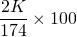The atomic weight or molecular weight is the relative weight of each atom compared to other atoms. The base element to which all other elements are measured is oxygen and it is given the value of 16.00. The atomic or molecular weights of the most important elements used in hydroponics are provided below. The values in brackets are rounded and are used in calculations since the level accuracy using the rounded values is adequate. The weight has a relative value but has a direct practical application as shall be seen in later sections.Macro- and micro element atomic weight which is used in the calculation of hydroponic fertilizer requirements.

The molecular weight of a molecule such as water (H2O) or potassium sulphate (K2SO4) can be determined by added the atomic weights of all the elements together. For instance water (H2O) will be:

(H × 2) + O = (1 × 2) + 16 = 18

The molecular weight of potassium sulphate (K2SO4) is calculated as follows:

(K×2)+S+(O×4) = (39×2)+32+(16×4) = 174

In order to calculate what percentage of potassium sulphate (K2SO4) consists of potassium the following procedure is followed:=44.83%

The following statement might be difficult to understand but it comes in very handy in calculating nutrient formulations. If there is 44.83 % potassium in potassium sulphate (K2SO4), it implies that there is 44.83 units of potassium in every 100 units of potassium sulphate. That is what a percentage is. The units can be anything from grams to kilograms. Take note of the following statements:

There is 44.83 units K in 100 units of potassium sulphate

There is 44.83 grams K in 100 grams potassium sulphate

There is 44.83 kilogram K in 100 kilogram potassium sulphate

There is 44.83 mg K in 100 mg potassium sulphate

Note that the units always stay the same. Many people make the mistake by mixing units of measure. Example:

There is 44.83 g K in 100 kg of potassium sulphate (WRONG!!)

The correct values are:

There is 44,830.00 g K in 100 kg of potassium sulphate (CORRECT!!)

Remember there is 1,000 g in 1 kg, thus the amount of potassium must be multiplied by 1,000 if measured in grams and potassium sulphate (K2SO4) is measured in kilograms.

The following unit are extensively used and the ratio’s to each other must be kept in mind when calculating formulations and mixing fertilizers.

• 1,000 µg = 1 mg
• 1,000 mg = 1 g
• 1,000 g = 1 kg
• 1,000 kg = 1 ton